# Publications

Here are some additional plots showing various aspects of a comparison of the performance of the old traversal scheme (red) and the new one (green):

Average Maximum
Object Obj name Time Num overlap tests Num DOP transforms Num nodes visited Num pgon intersection tests Time Num overlap tests Num DOP transforms Num nodes visited Num pgon intersection tests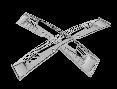Cover (Abdeckung), 30477 polygons each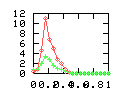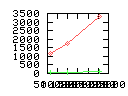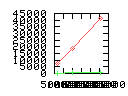Happy Buddha (buddha), 125,000 polygons each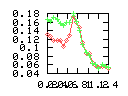Front Light (scheinwerfer), 30075 polygons each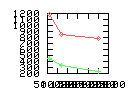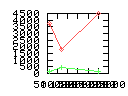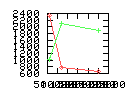Door Lock (schloss), 26136 polygons eachCar Body (sharan), 28167 polygons eachThe time plots show the collision detection time depending on the "distance" between the two objects, for one polygon count (as given in the column "obj name"). The "num" plots show statistics of various characteristic numbers (see below for an explanation), depending on the polygon count.

Benchmarking procedure: two identical objects are positioned at a certain distance d = dstart from each other. The distance is computed between the centers of the bounding boxes of the two objects; objects are scaled uniformly so they fit in a cube of size [-1,+1]3. Then, one of them performs a full tumbling turn about the z- and the x-axis by a fixed, large number of small steps (5000). With each step, a collision query is done, and the average collision detection time for a complete revolution at that distance is computed. Then, d is decreased, and a new average collision detection time is computed. The collision detection algorithm always stopped whenever the first pair of intersecting polygons was found.

Explanation of the columns:

• Time: average collision detection time for a certain distance; the average was taken over many collision queries with different object orientations (see above).

• Num overlap tests: average number of BV pair overlap tests during one collision test.

• Num DOP transforms: average number of DOP transformations that are performed during one collision test.

• Num nodes visited: total average number of BV tree nodes that are visited during one collision test; this counts both tree's nodes, and multiple visits of the same node are counted multiple times. So, this number is proportional to the amount of data that must be transfered from memory during collision test.

• Num pgon intersection tests: average number of polygon-polygon intersection tests; this is the number of leaf pairs that are reached during one collision test without finding a collision.

The maxium columns are exactly the same as the average, except that here, the maximum over all rotations (with one distance) is listed.